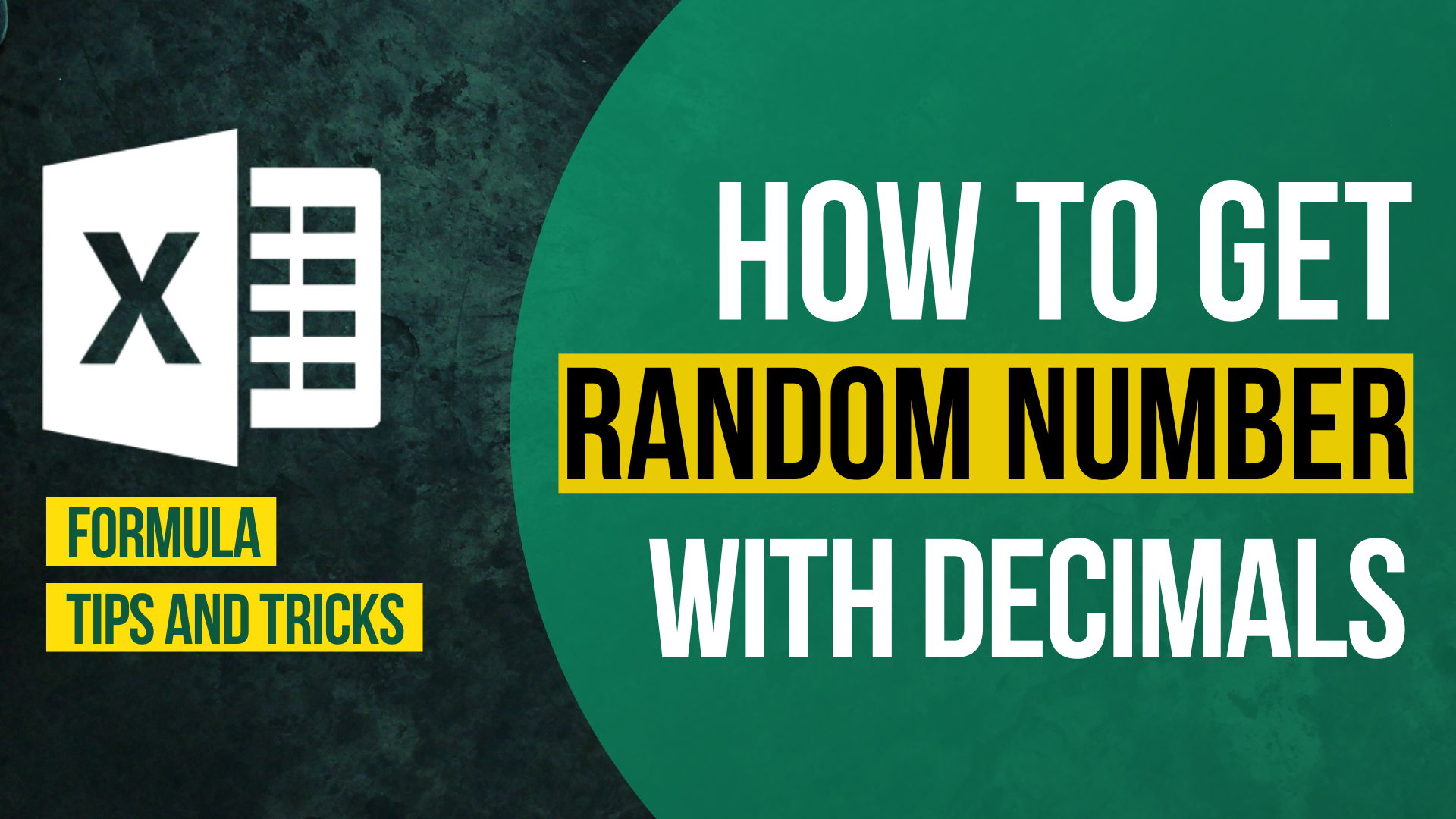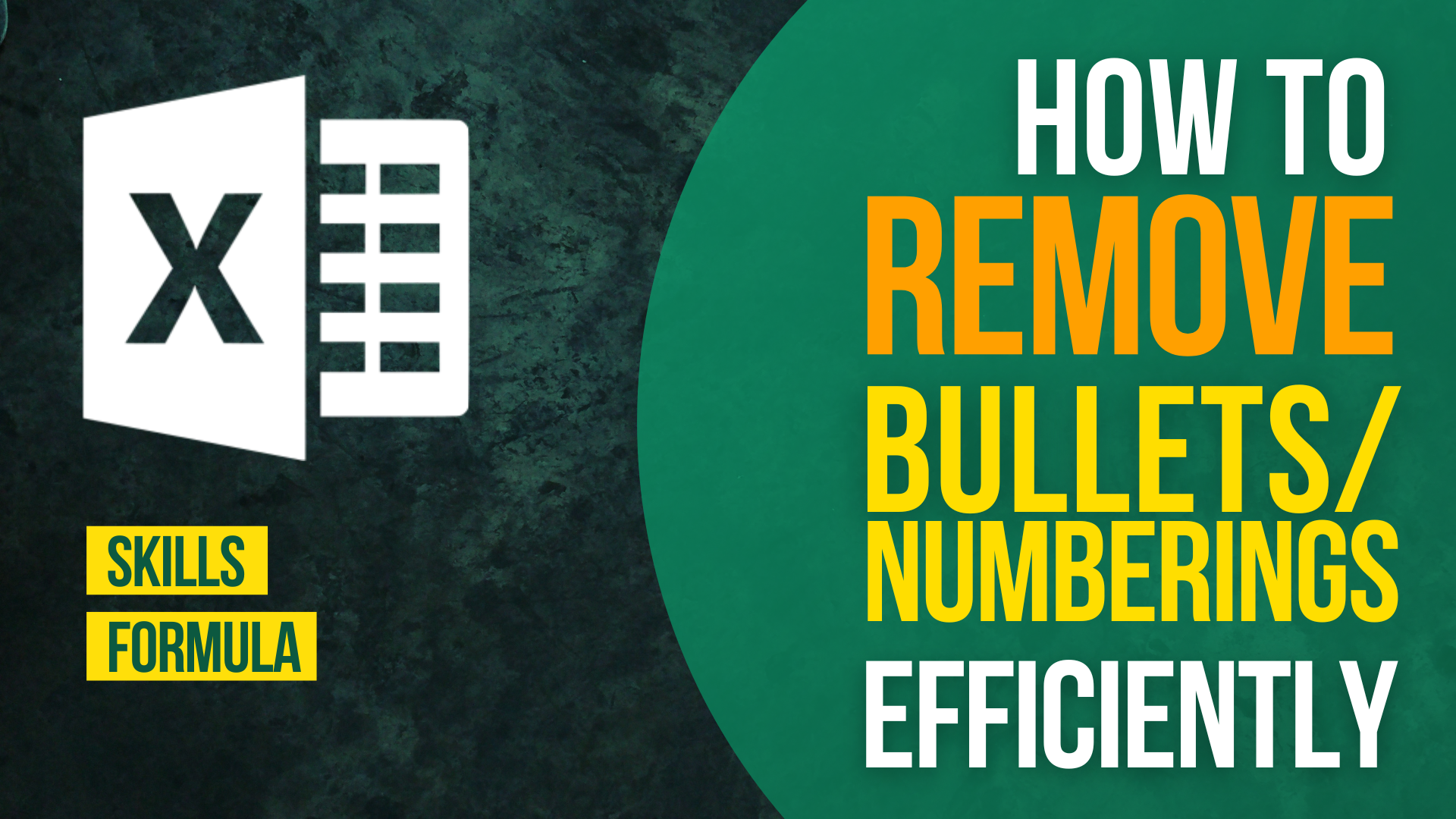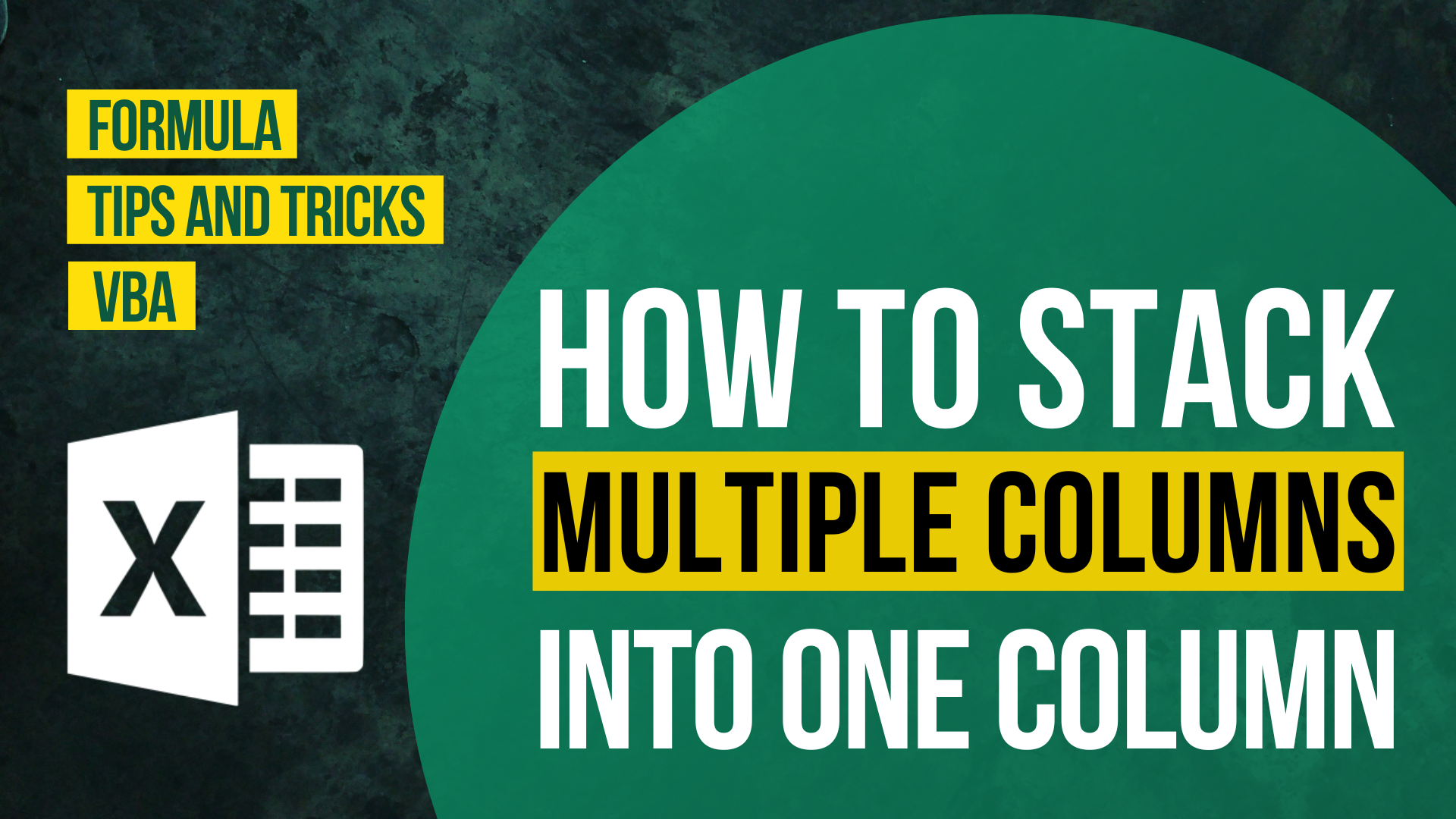# How to Generate Random Numbers with DecimalsGenerating random numbers has always been a big topic in Excel. Its usage includes but does not limit to dividing people into different groups, lot drawings, sampling. Generating integer is relatively easy with the introduction of RANDBETWEEN function.

However, there isn’t a function that allows you to get random numbers with decimals.

In this article, I will show you how to get random numbers with decimals in Excel. There are 3 formulae of your choice to cope with different situation.

If you have the following questions, I suggest you reading this until the end.

• How to use Randbetween() to return decimals in Excel?
• How to generate random decimals in Excel?
• How to get random number with decimal place in Excel?

You might also be interested in Excel Split Long Text into Short Cell Without Splitting Word.

## Introducing Randbetween()

Randbetween() is frown upon by many Excel users when it comes to generating random numbers with decimal.

Let’s take a look at how Microsoft describe Randbetween function.

While Randbetween() is a fantastic function to generate a random number between the specified numbers, there is a major drawback of using Randbetween(). It cannot generate random decimals.

No worries. I will show you how to use Randbetween() to generate random decimals.

You might also be interested in How to prevent duplicate entries in Excel?

## Formula 1: Generate random numbers with decimals in Excel by Randbetween() (Simplest)

### What we are trying to do

`To generate random number between 50 to 80 with 2 decimal places`

Using Randbetween() the traditional way, we would have got random integer like 62, 71 or so. Therefore, we are going to twist the formula a bit.

Among 3 formulae, formula is the simplest one. It is most suitable for one-off usage. For instance, when you are presenting in front of your boss and you want to impress him by your Excel skilI and speed. If you are not likely to change the formula and won’t bother by the rigidity of the formula, then you may enjoy this the most.

If you are looking for a more flexible formula which allows you to change the input easily, I suggest you jump to another section.

### Formula in cell A6

`=RANDBETWEEN(5000,8000)/1000`

### How does it work

Instead of putting 50 and 80, we multiply them by 100 and put 5000 and 8000 here. This is because Randbetween(5000,8000) will result in a 4-digit number. By dividing the 4-digit number by 100, we will get a 2 digit number with 2 decimal places.

Take cell A6 as an example, Randbetween(5000,6000) result in 6819. We then divide 6819 by 100 and get 68.19.

### How to control the number of decimal places

You may wonder how to control the number of decimal places. It is indeed very easy.

Number of decimal places = Number of zero in the number you multiply by (it has to be in multiples of 10)

#### More examples

Let’s say I would like to get a random number between 489 and 762 with 4 decimal places. The formula would have been this.

`=RANDBETWEEN(4890000,7620000)/10000`

The result would look like this.

Let’s say I would like to get a random number between 2 and 5 with 2 decimal places. The formula would have been this.

`=RANDBETWEEN(200,500)/100`

## Formula 2: Generate random numbers with decimals in Excel by Randbetween()

### What we are trying to do

`To generate random number between 50 to 80 with 2 decimal places`

What I am going to do is to twist the previous formula a bit and make it more flexible. This is like a little facelift of the formula 1.

### Formula in cell A6

`=RANDBETWEEN(50*100, 80*100) /100`

The difference between formula 1 and 2 is very small. In fact we merely gave the formula more flexibility. The formula is also more intuitive and easy to read.

### How does it work

Instead of putting 50 and 80, we put 50*100 and 80*100 . This is because Randbetween(5000,8000) will result in a 4-digit number. By dividing the 4-digit number by 100, we will get a 2 digit number with 2 decimal places.

Take cell A6 as an example, Randbetween(5000,8000) result in 5503. We then divide 5503 by 100 and get 55.03.

### How to control the number of decimal places

You may wonder how to control the number of decimal places. It is indeed very easy.

Number of decimal places = Number of zero in the number you multiply by (it has to be in multiples of 10)

#### More examples

Let’s say I would like to get a random number between 489 and 762 with 4 decimal places. The formula would have been this.

`=RANDBETWEEN(489*10000,762*10000)/10000`

The result would look like this.

Let’s say I would like to get a random number between 2 and 5 with 2 decimal places. The formula would have been this.

`=RANDBETWEEN(2*100,5*100)/100`

## Formula 3: Generate random numbers with decimals in Excel by Randbetween() and Rand() (most flexible)

### What we are trying to do

`To generate random number between 50 to 80 with 2 decimal places`

If you are looking for a highly flexible formula which is easy to edit, the formula 3 is a tailor-made for you.

### Formula in cell A6

`=RANDBETWEEN(B2,D2)+(ROUND((RAND()),2))`

### How does it work

`RANDBETWEEN(B2,D2)`

The former part of the formula is easy to understand. It helps generating a random integer between the specified value (50 and 80).

`(ROUND((RAND()),2))`

The latter part of the formula is also intuitive. RAND() helps generating a decimal which is smaller than 1 and ROUND() rounds the decimal to the specified number of decimal places. Here we round the number to 2 decimal places.

When the former part and the latter part adds up, they result in a number between 50 and 80 with 2 decimal places.

### How to control the number of decimal places

You would like to change this part.

`(ROUND((RAND()),number of decimal places))`

#### More examples

Let’s say I would like to get a random number between 489 and 762 with 4 decimal places. The formula would have been this.

Input the value of B2 and D2 as 489 and 762 respectively.

`=RANDBETWEEN(B2,D2)+(ROUND((RAND()),4))`

The result would look like this.

Let’s say I would like to get a random number between 2 and 5 with 2 decimal places. The formula would have been this.

Input the value of B2 and D2 as 489 and 762 respectively.

`=RANDBETWEEN(B2,D2)+(ROUND((RAND()),2))`

#### Why formula 3 is the most flexible solution among 3

You may notice that you only need to change 1 variable when editing formula 3 This is what we call flexibility of formula in Excel. A flexible formula allows you to change the variable easily and less prone to mistake.

## Why some cells contains less decimal places

Even we applied the same formula to a table of cells, some cells contain less decimal places than the others. This is not an error. Instead, this is because those cells happens to 61.40, 79,00 and 70.80. Excel normally hide the 0 after the last non-zero decimal places.

If you would like to show the decimal places, you can twist the number format.

If you are not content with some cells not being a actual decimal, you can try to force the Excel sheet to recalculate. The shortcut to recalculate the Excel sheet is F9.

You might also be interested in How to Generate Random Dates in Excel?

Hungry for more useful Excel tips like this? Subscribe to our newsletter to make sure you won’t miss out on any of our posts and get exclusive Excel tips!How to Input Time Duration in ExcelHow to Remove Bullets/Numberings in Excel

Popular posts